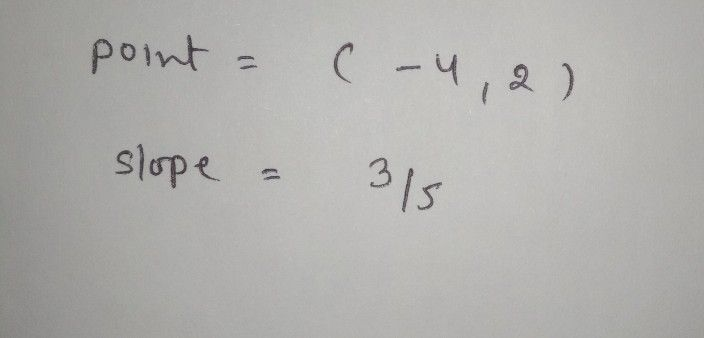Symbol
ProblemQUESTION $2$ Use the following linear equation: $y+4=$ $frac|\left(3\right)\left(53\left(x-2\right)$ Point $=\left($ $S$ Slope $=$
10th-13th grade
Algebra
Question content
This is from a algebra and trig class .. please make sure hand writing is good
SolutionQanda teacher - yadav505hey dear feel free to ask queries
please evaluate the answer if you satisfied
Please give 3000 coins as thank you gift this will not reduce your coins
happy learning ☺️☺️Student
If I wanted to graph this equation what will the points be on the graph ?